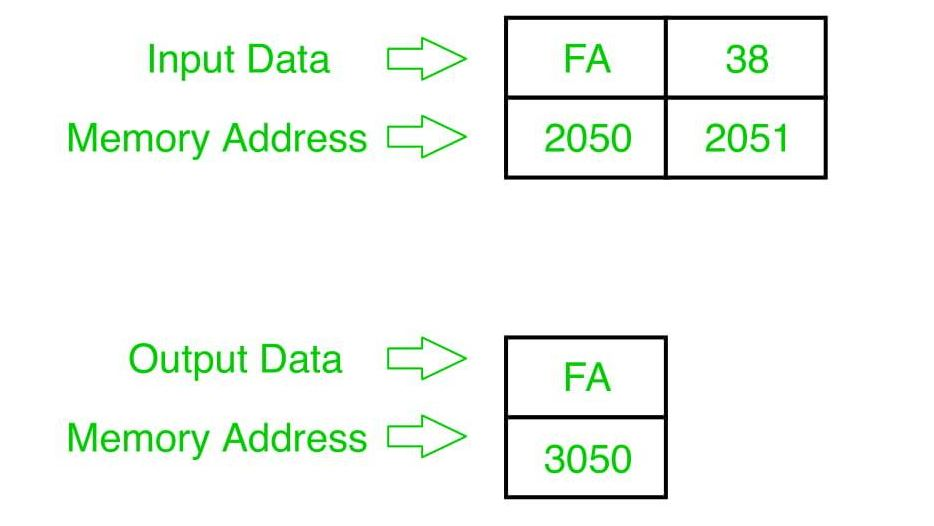# 8085 program to find larger of two 8 bit numbers

• Last Updated : 22 May, 2018

Problem – Write a program in 8085 microprocessor to find out larger of two 8-bit numbers, where numbers are stored in memory address 2050 and 2051, and store the result into memory address 3050.

Example –Algorithm –

1. Load two numbers from memory 2050 & 2051 to register L and H .
2. Move one number(H) to Accumulator A and subtract other number(L) from it.
3. if result is positive then move the number(H) to A and store value of A at memory address 3050 and stop else move the number(L) to A and store value of A at memory address 3050 and stop.

Program –

2000 LHLD 2050 H<-(data at 2051)&L<-(data at 2050)
2003 MOV A, H A<-H
2004 SUB L A<-A-L
2008 MOV A, L A<-L
2009 STA 3050 A->(in memory 3050)
200C HLT STOP
200D MOV A, H A<-H
200E STA 3050 A->(in memory 3050)
2011 HLT STOP

Explanation –

1. LHLD 2050: load data from memory 2050 & 2051 to register L and H.
2. MOV A, H: transfer contents of register H to A.
3. SUB L: subtract contents of register L from A and store it to A.